Courses

# Calculus (Part - 2) - Civil Engineering Civil Engineering (CE) Notes | EduRev

## Topic wise GATE Past Year Papers for Civil Engineering

Created by: Gate Gurus

## Civil Engineering (CE) : Calculus (Part - 2) - Civil Engineering Civil Engineering (CE) Notes | EduRev

The document Calculus (Part - 2) - Civil Engineering Civil Engineering (CE) Notes | EduRev is a part of the Civil Engineering (CE) Course Topic wise GATE Past Year Papers for Civil Engineering.
All you need of Civil Engineering (CE) at this link: Civil Engineering (CE)

Question 16:[2017 : 1 Mark, Set-I]
Solution: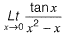(Applying L'Hospital rule)

=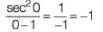Question 17: The quadratic approximation of
f(x) = x3 - 3x2 - 5 a the point x = 0 is    [2016 : 2 Marks, Set-II]
(a) 3x2 - 6x - 5
(b) -3x2 - 5
(c) -3x2 + 6x - 5
(d) 3x2 - 5
Solution: The quadratic approximation of f{x) at the point x = 0 is,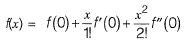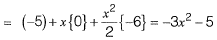Question 18: The area between the parabola x2 = 8y and the straight line y = 8 is______.    [2016 : 2 Marks, Set-II]
Solution: Parabola is x2 = 8y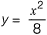and straight is y = 0
At the point of intersection, we have,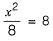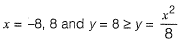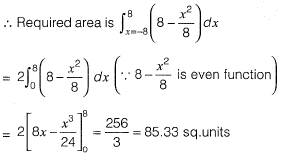Question 19: The angle of intersection of the curves x2 = 4y and y2 = 4x at point (0, 0) is     [2016 : 2 Marks, Set-II]
(a) 0°
(b) 30°
(c) 45°
(d) 90°
Solution: Given curve,
x2 = 4y     .......(i)
and y2 = 4x    ........(ii)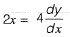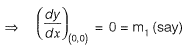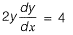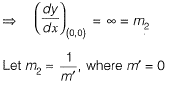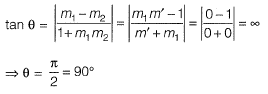Question 20: The area of the region bounded by the parabola y = x2 + 1 and the straight line x + y = 3 is
(a) 59/6
(b) 9/2
(c) 10/3
(d) 7/6
Solution: At the point of intersection of the curves,
y = x2 + 1 and x + y = 3 i.e., y = 3 - x , we have,
x2 + 1 - 3 - x ⇒ x2 + x - 2 = 0
⇒ x = -2, 1 and 3 - x > x2 + 1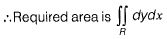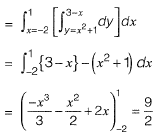Question 21: The value of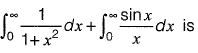[2016 : 2 Marks, Set-I]
(a) π/2
(b) π
(c) 3π/2
(d) 1
Solution: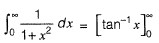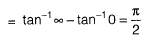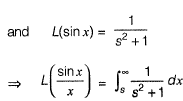(Using "division by x")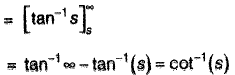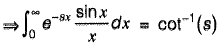(Using definition of Laplace transform)
Put s - 0, we get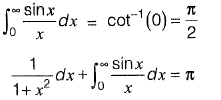Question 22: What is the value of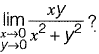[2016 : 1 Mark, Set-II]
(a) 1
(b) -1
(c) 0
(d) Limit does not exit
Solution: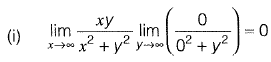(i.e., put x = 0 and then y = 0)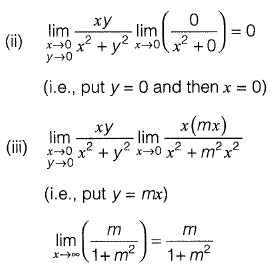which depends on m.

Question 23: The optimum value of the function f(x) = x2 - 4x + 2 is    [2016 : 1 Mark, Set-II]
(a) 2 (maximum)
(b) 2 (minimum)
(c) -2 (maximum)
(d) -2 (minimum)
Solution:
f'(x) = 0
⇒ 2x — 4 = 0
x = 2 (stationary point)
f"(x) = 2 > 0

⇒ f(x) is minimum at x = ?
i.e., (2)2 - 4(2) + 2 = -2
∴ The optimum value of f(x) is -2 (minimum)

Question 24: While minimizing the function f(x), necessary and sufficient conditions for a point x0 to be a minima are    [2015 : 1 Mark, Set-II]
(a) f' (x0) > 0 and f" (x0) = 0
(b) f'(x0)< 0 an d f"(x0) = 0
(c) f' (x0) = 0 and f" (x0) < 0
(d) f' (x0) = 0 and f" (x0) > 0
Solution: f(x) has a local minimum at x = x0
if f'(x0) = 0 and f''(x0) > 0

Question 25: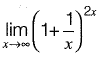is equal to    [2015 : 1 Mark, Set-II]
(a) e-2
(b) e
(c) 1
(d) e2
Solution: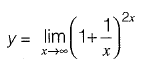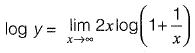Which is in the form of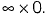To convert this into 0/0  form, we rewrite as,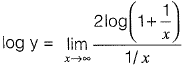Now it is in 0/0 form.
Using L’Hospital’s rule,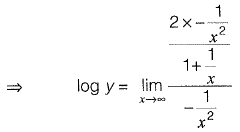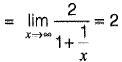∴ y = e2

Question 26: The directional derivative of the field u(x, y, z) = x2 - 3yz in the direction of the vector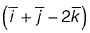at point (2, - 1, 4) is _________.    [2015 : 2 Marks, Set-I]
Solution: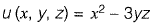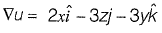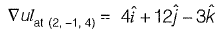Directional derivative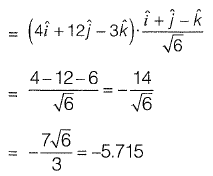Question 27: The expression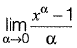is equal to    [2014 : 2 Marks, Set-II]
(a) ln x
(b) 0
(c) x ln x
(d) ∞
Solution: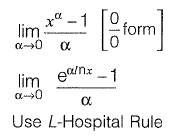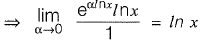Question 28: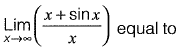[2014 : 1 Marks, Set-I]
(a) -
(b) 0
(c) 1
(d) ∞
Solution: Put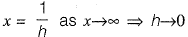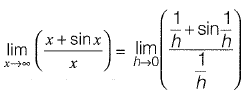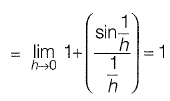Question 29: The value of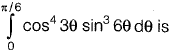[2013 : 2 Marks]
(a) 0
(b) 1/15
(c) 1
(d) 8/3
Solution: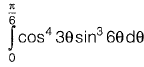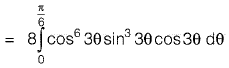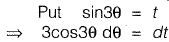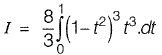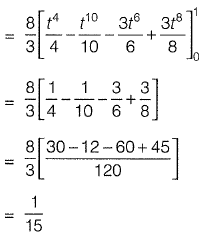Alternative Method: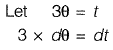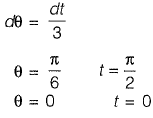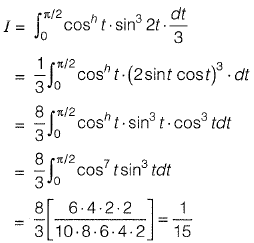Question 30: For the parallelogram OPQR shown in the sketch,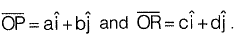The area of the parallelogram is    [2011 : 2 Marks]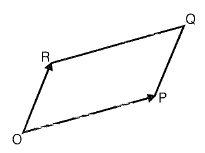(b) ac + bd
(d) ab - cd
Solution: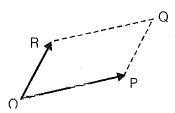The area of parallelogram OPQR in figure shown above, is the magnitude of the vector product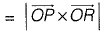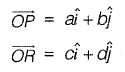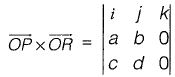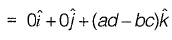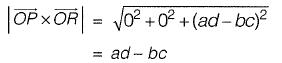,

,

,

,

,

,

,

,

,

,

,

,

,

,

,

,

,

,

,

,

,

;